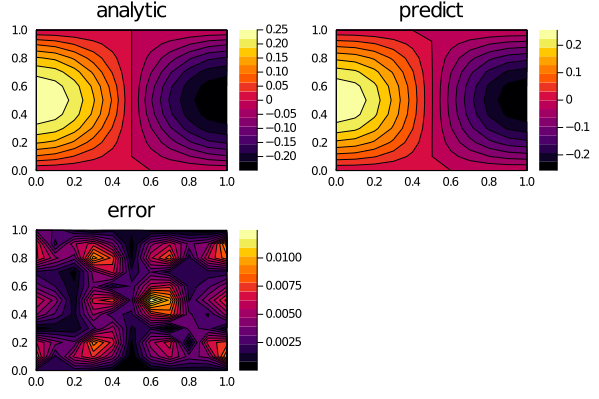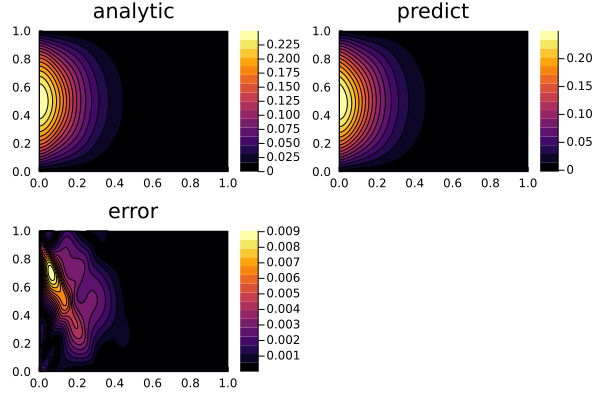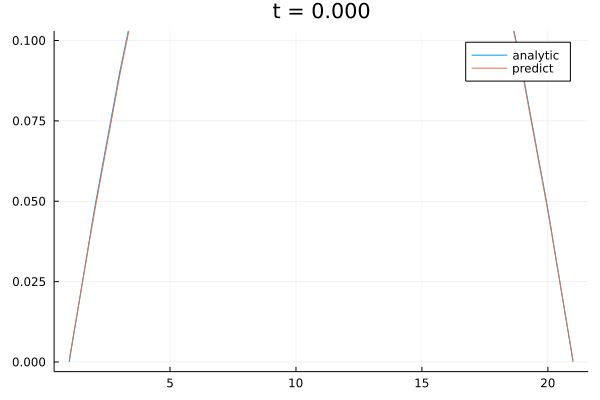# 1D Wave Equation with Dirichlet boundary conditions

Let's solve this 1-dimensional wave equation:

\begin{align*} ∂^2_t u(x, t) = c^2 ∂^2_x u(x, t) \quad & \textsf{for all } 0 < x < 1 \text{ and } t > 0 \, , \\ u(0, t) = u(1, t) = 0 \quad & \textsf{for all } t > 0 \, , \\ u(x, 0) = x (1-x) \quad & \textsf{for all } 0 < x < 1 \, , \\ ∂_t u(x, 0) = 0 \quad & \textsf{for all } 0 < x < 1 \, , \\ \end{align*}

with grid discretization dx = 0.1 and physics-informed neural networks.

Further, the solution of this equation with the given boundary conditions is presented.

using NeuralPDE, Flux, ModelingToolkit, Optimization, GalacticOptimJL, DiffEqFlux
import ModelingToolkit: Interval, infimum, supremum

@parameters t, x
@variables u(..)
Dxx = Differential(x)^2
Dtt = Differential(t)^2
Dt = Differential(t)

#2D PDE
C=1
eq  = Dtt(u(t,x)) ~ C^2*Dxx(u(t,x))

# Initial and boundary conditions
bcs = [u(t,0) ~ 0.,# for all t > 0
u(t,1) ~ 0.,# for all t > 0
u(0,x) ~ x*(1. - x), #for all 0 < x < 1
Dt(u(0,x)) ~ 0. ] #for all  0 < x < 1]

# Space and time domains
domains = [t ∈ Interval(0.0,1.0),
x ∈ Interval(0.0,1.0)]
# Discretization
dx = 0.1

# Neural network
chain = FastChain(FastDense(2,16,Flux.σ),FastDense(16,16,Flux.σ),FastDense(16,1))
initθ = Float64.(DiffEqFlux.initial_params(chain))
discretization = PhysicsInformedNN(chain, GridTraining(dx); init_params = initθ)

@named pde_system = PDESystem(eq,bcs,domains,[t,x],[u(t,x)])
prob = discretize(pde_system,discretization)

callback = function (p,l)
println("Current loss is: l") return false end # optimizer opt = GalacticOptimJL.BFGS() res = Optimization.solve(prob,opt; callback = callback, maxiters=1200) phi = discretization.phi We can plot the predicted solution of the PDE and compare it with the analytical solution in order to plot the relative error. using Plots ts,xs = [infimum(d.domain):dx:supremum(d.domain) for d in domains] analytic_sol_func(t,x) = sum([(8/(k^3*pi^3)) * sin(k*pi*x)*cos(C*k*pi*t) for k in 1:2:50000]) u_predict = reshape([first(phi([t,x],res.minimizer)) for t in ts for x in xs],(length(ts),length(xs))) u_real = reshape([analytic_sol_func(t,x) for t in ts for x in xs], (length(ts),length(xs))) diff_u = abs.(u_predict .- u_real) p1 = plot(ts, xs, u_real, linetype=:contourf,title = "analytic"); p2 =plot(ts, xs, u_predict, linetype=:contourf,title = "predict"); p3 = plot(ts, xs, diff_u,linetype=:contourf,title = "error"); plot(p1,p2,p3)## 1D Damped Wave Equation with Dirichlet boundary conditions Now let's solve the 1-dimensional wave equation with damping. \begin{aligned} \frac{\partial^2 u(t,x)}{\partial x^2} = \frac{1}{c^2} \frac{\partial^2 u(t,x)}{\partial t^2} + v \frac{\partial u(t,x)}{\partial t} \\ u(t, 0) = u(t, L) = 0 \\ u(0, x) = x(1-x) \\ u_t(0, x) = 1 - 2x \\ \end{aligned} with grid discretization dx = 0.05 and physics-informed neural networks. Here we take advantage of adaptive derivative to increase accuracy. using NeuralPDE, Flux, ModelingToolkit, Optimization, GalacticOptimJL, DiffEqFlux using Plots, Printf import ModelingToolkit: Interval, infimum, supremum @parameters t, x @variables u(..) Dxu(..) Dtu(..) O1(..) O2(..) Dxx = Differential(x)^2 Dtt = Differential(t)^2 Dx = Differential(x) Dt = Differential(t) # Constants v = 3 b = 2 L = 1.0 @assert b > 0 && b < 2π / (L * v) # 1D damped wave eq = Dx(Dxu(t, x)) ~ 1 / v^2 * Dt(Dtu(t, x)) + b * Dtu(t, x) # Initial and boundary conditions bcs_ = [u(t, 0) ~ 0.,# for all t > 0 u(t, L) ~ 0.,# for all t > 0 u(0, x) ~ x * (1. - x), # for all 0 < x < 1 Dtu(0, x) ~ 1 - 2x # for all 0 < x < 1 ] ep = (cbrt(eps(eltype(Float64))))^2 / 6 der = [Dxu(t, x) ~ Dx(u(t, x)) + ep * O1(t, x), Dtu(t, x) ~ Dt(u(t, x)) + ep * O2(t, x)] bcs = [bcs_;der] # Space and time domains domains = [t ∈ Interval(0.0, L), x ∈ Interval(0.0, L)] # Neural network inn = 25 innd = 4 chain = [[FastChain(FastDense(2, inn, Flux.tanh), FastDense(inn, inn, Flux.tanh), FastDense(inn, inn, Flux.tanh), FastDense(inn, 1)) for _ in 1:3]; [FastChain(FastDense(2, innd, Flux.tanh), FastDense(innd, 1)) for _ in 1:2];] initθ = map(c -> Float64.(c), DiffEqFlux.initial_params.(chain)) strategy = GridTraining(0.02) discretization = PhysicsInformedNN(chain, strategy; init_params=initθ) @named pde_system = PDESystem(eq, bcs, domains, [t, x], [u(t, x), Dxu(t, x), Dtu(t, x), O1(t, x), O2(t, x)]) prob = discretize(pde_system, discretization) pde_inner_loss_functions = prob.f.f.loss_function.pde_loss_function.pde_loss_functions.contents inner_loss_functions = prob.f.f.loss_function.bcs_loss_function.bc_loss_functions.contents bcs_inner_loss_functions = inner_loss_functions callback = function (p, l) println("Current loss is:l")
println("pde_losses: ", map(l_ -> l_(p), pde_inner_loss_functions))
println("bcs_losses: ", map(l_ -> l_(p), bcs_inner_loss_functions))
return false
end

res = Optimization.solve(prob, BFGS();callback = callback, maxiters=2000)
prob = remake(prob, u0=res.minimizer)
res = Optimization.solve(prob, BFGS();callback = callback, maxiters=2000)

phi = discretization.phi

# Analysis
ts, xs = [infimum(d.domain):0.05:supremum(d.domain) for d in domains]

μ_n(k) = (v * sqrt(4 * k^2 * π^2 - b^2 * L^2 * v^2)) / (2 * L)
b_n(k) = 2 / L * -(L^2 * ((2 * π * L - π) * k * sin(π * k) + ((π^2 - π^2 * L) * k^2 + 2 * L) * cos(π * k) - 2 * L)) / (π^3 * k^3) # vegas((x, ϕ) -> ϕ = sin(k * π * x) * f(x)).integral
a_n(k) = 2 / -(L * μ_n(k)) * (L * (((2 * π * L^2 - π * L) * b * k * sin(π * k) + ((π^2 * L - π^2 * L^2) * b * k^2 + 2 * L^2 * b) * cos(π * k) - 2 * L^2 * b) * v^2 + 4 * π * L * k * sin(π * k) + (2 * π^2 - 4 * π^2 * L) * k^2 * cos(π * k) - 2 * π^2 * k^2)) / (2 * π^3 * k^3)

# Plot
analytic_sol_func(t,x) = sum([sin((k * π * x) / L) * exp(-v^2 * b * t / 2) * (a_n(k) * sin(μ_n(k) * t) + b_n(k) * cos(μ_n(k) * t)) for k in 1:2:100]) # TODO replace 10 with 500
anim = @animate for t ∈ ts
@info "Time \$t..."
sol =  [analytic_sol_func(t, x) for x in xs]
sol_p =  [first(phi([t,x], res.minimizer)) for x in xs]
plot(sol, label="analytic", ylims=[0, 0.1])
title = @sprintf("t = %.3f", t)
plot!(sol_p, label="predict", ylims=[0, 0.1], title=title)
end

# Surface plot
ts, xs = [infimum(d.domain):0.01:supremum(d.domain) for d in domains]
u_predict = reshape([first(phi([t,x], res.minimizer)) for t in ts for x in xs], (length(ts), length(xs)))
u_real = reshape([analytic_sol_func(t, x) for t in ts for x in xs], (length(ts), length(xs)))

diff_u = abs.(u_predict .- u_real)
p1 = plot(ts, xs, u_real, linetype=:contourf, title="analytic");
p2 = plot(ts, xs, u_predict, linetype=:contourf, title="predict");
p3 = plot(ts, xs, diff_u, linetype=:contourf, title="error");
plot(p1,p2,p3)

We can see the results here:Plotted as a line one can see the analytical solution and the prediction here: